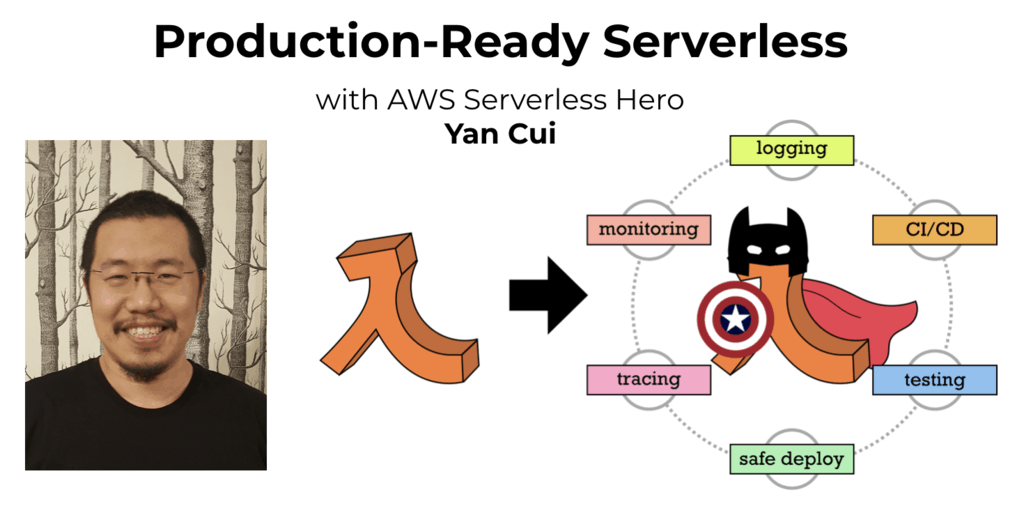# Project Euler – Problem 34 Solution

Check out my new course Learn you some Lambda best practice for great good! and learn the best practices for performance, cost, security, resilience, observability and scalability.

#### Problem

145 is a curious number, as 1! + 4! + 5! = 1 + 24 + 120 = 145.

Find the sum of all numbers which are equal to the sum of the factorial of their digits.

Note: as 1! = 1 and 2! = 2 are not sums they are not included.

#### Solution

```open System.Collections.Generic

// factorial function
let factorial n = if (n = 0) then 1 else [1..n] |> List.reduce (fun acc x -> acc * x)

// create a cache of sorts to help improve performance
let factorials = new Dictionary<int, int>()

let start = [0..9] |> List.iter (fun n -> factorials.Add(n, (factorial n)))

// get the digits of a number into an array
let getDigits (n:bigint) =
n.ToString().ToCharArray()
|> Array.map (fun c -> int(c.ToString()))

// get the sum of the factorials of a number's digits
let getDigitsToFactSum (n:bigint) =
bigint(getDigits(n) |> Array.map (fun n -> factorials.[n]) |> Array.sum)

// get the max sum achievable by a number of the given number of digits
let upperBound (digits:int) = bigint(digits) * bigint(factorial 9)

// find the last number of digits n, where the max sum achievable by the factorials of the
// digits of a number of n digits is greater than the smallest number of n digits
// any number with more than n digits do not need to be checked
let digitsToCheck =
let n =
Seq.unfold (fun state -> Some(state, (state+1))) 1
|> Seq.filter (fun x -> (upperBound x).ToString().ToCharArray().Length < x)
n-1

// get the next number with the given number of digits
let maxNumber digits = [1..digits] |> List.map (fun x -> 9I * pown 10I (x-1)) |> List.sum

let max = maxNumber digitsToCheck
[3I..max] |> List.filter (fun n -> n = getDigitsToFactSum n) |> List.sum
```

This solution is very similar to my solution for problem 30, with the main difference being that the sum is calculated using the factorials of the digits rather the fifth power.Hi, I’m Yan. I’m an AWS Serverless Hero and the author of Production-Ready Serverless.

I specialise in rapidly transitioning teams to serverless and building production-ready services on AWS.

Are you struggling with serverless or need guidance on best practices? Do you want someone to review your architecture and help you avoid costly mistakes down the line? Whatever the case, I’m here to help.Check out my new course, Learn you some Lambda best practice for great good! In this course, you will learn best practices for working with AWS Lambda in terms of performance, cost, security, scalability, resilience and observability. Enrol now and enjoy a special preorder price of £9.99 (~\$13).Are you working with Serverless and looking for expert training to level-up your skills? Or are you looking for a solid foundation to start from? Look no further, register for my Production-Ready Serverless workshop to learn how to build production-grade Serverless applications!